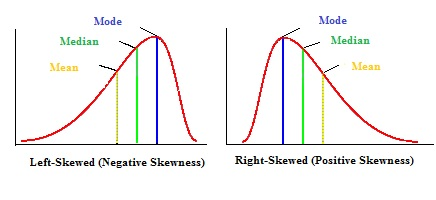# Review 1

## Measures of central tendency and measures of dispersion

### Mean, median, mode

Median: The median is the middle value of the ordered sample values. It is the number which is half-way into the list of reported values

Mode: The mode is the value with highest frequency in the sample.

Mean:

$\overline{x} = \frac{1}{n}\sum x_i$

### Easier way to calculate the mean

Sample: 0, 0, 1, 3, 3, 4, 4, 4, 5, 8.

x f fx
0 2 0
1 1 1
3 2 6
4 3 12
5 1 5
8 1 8
$$\sum x = 21$$ $$\sum f=10$$ $$\sum fx=32$$

$\overline{x} = \frac{\sum fx}{\sum f} = \frac{32}{10} = 3.2$

### Skweness

It is important to understand how the skewness of a distribution influences the relationship between the mean, median and mode. The figure below is from here.### Standard deviation

$sd = \sqrt{\frac{f(x - \overline{x})^2}{n-1} }$

$$x$$ f fx $$x - \overline{x}$$ $$(x - \overline{x})^2$$ $$f(x - \overline{x})^2$$
. $$\sum f$$ $$\sum fx$$ $$\sum above = A$$

Calculate the standard deviation: $sd = \sqrt{\frac{A}{n-1} }$

### Variance

$variance = sd^2$

## Z-scores

$z=\frac{x_i-\overline{x}}{sd}$

where $$s$$ is the sample standard deviation

Recall that, for the z-distribution:

• Mean = 0
• SD = 1

## Confidence intervals

Standard error: $se = \frac{sd}{\sqrt{n}}$

CIs: $\text{Lower bound} = \bar x - z_{(\frac{\alpha}{2})}se$

$\text{Upper bound} = \bar x + z_{(\frac{\alpha}{2})}se$

• For 95% CI: $$z_{(\frac{\alpha}{2})}= z_{0.025} \approx 2$$
• For 99% CI: $$z_{(\frac{\alpha}{2})}= z_{0.005} \approx 3$$

## Hypothesis testing: one-sample t-test

$t = \frac {\bar x-\mu_0}{se}$

1. We reject the null hypothesis only when the calculated $$t$$ is higher than the critical value ($$c_\alpha$$).
1. We reject the null hypothesis only when $$p \leq \alpha$$
1. We reject the null hypothesis if the mean difference CI does not include 0.

## Practice questions

1. Suppose $$\overline{x} = 10$$ for a negatively skewed distribution. What could be the median and mode of this distribution?
1. median = 11 and mode = 12
2. median = 12 and mode = 11
1. Suppose you created a z-score distribution from a given sample. What is the mean of this distribution?
1. 1
2. 0
3. There is not enough information to calculate the mean.
1. The p-value equals:
1. $$\alpha$$
2. The significance level
3. 0.05
1. What is the percentile of a z-score $$z= 1.18$$
1. 45%
2. 17%
3. 88%
1. Using the z-table, find the values of z:
1. That is just above 97.5% of all values in the distribution.
2. That is just above 99.5% of all values in the distribution.
1. Using the t-table, find the value of t for:
1. Two-tail, $$\alpha=0.05$$ (n=31)
2. One-tail, $$\alpha=0.05$$ (n=31)
3. Two-tail, $$\alpha=0.01$$ (n=31)
4. One-tail, $$\alpha=0.01$$ (n=31)
1. Compared to a 95% confidence interval, a 99% confidence interval:
1. Is more precise
2. Is more accurate
1. If $$p = 0.03$$
1. We reject the null when $$\alpha = 0.05$$
2. We reject the null when $$\alpha = 0.01$$
3. We never reject the null
1. As the absolute value of $$t_{calculated}$$ increases, we are:
1. More likely to reject the null
2. Less likely to reject the null
1. Given a 95% mean difference CI = (-0.12;1.2), do we reject the null that the population mean = 15 (assuming $$\alpha = 0.05)$$?
1. Yes
2. No
1. As sample size increases a 95% CI becomes:
1. Narrower, more precise
2. Less accurate